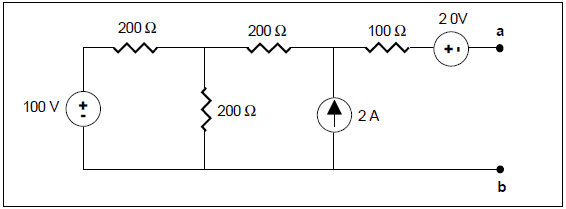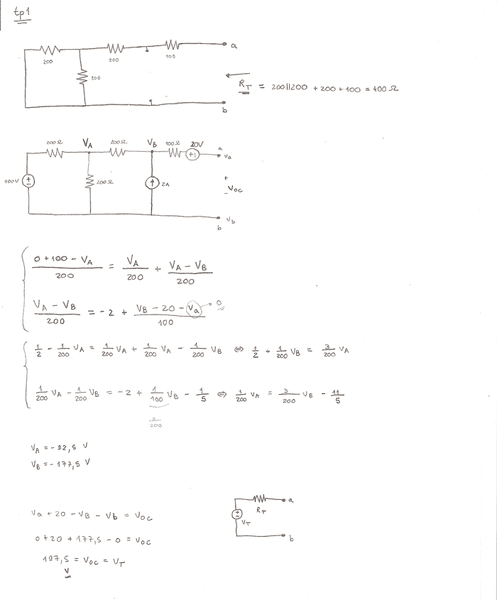# Thevenin and Norton Equivalent Circuits

• Engineering

## Homework Statement

Consider this circuit. Find the Thévenin and Norton Equivalent Circuits (just need the 1st one).## The Attempt at a Solution

My attempt:Can someone check? Thanks a lot!

ehild
Homework Helper
The Thevenin-equivalent resistance is correct.
You need to find the open-circuit voltage between a an b. If it is open no current flows through the 100 ohm resistor, so all the 2A current of the current generator flows to B and then to A, through the 200 ohm resistor. So you know at VB-VA.

ehild

So, there's an incorrect equation, right?

ehild
Homework Helper
Yes, the second one.

ehild

Is it just (VB-VA)/200=2?

ehild
Homework Helper
Yes.

ehild

Thanks a lot!